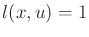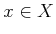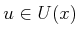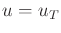#### Dial's algorithm

Now consider generalizing the wavefront propagation idea. Wavefront propagation can be applied to any discrete planning problem iffor anyand(except). It is most useful when the transition graph is sparse (imagine representing the transition graph using an adjacency matrix). The grid problem is a perfect example where this becomes important. More generally, if the cost terms assume integer values, then Dial's algorithm  results, which is a generalization of wavefront propagation, and a specialization of Dijkstra's algorithm. The idea is that the priority queue can be avoided by assigning the alive vertices to buckets that correspond to different possible cost-to-go values. In the wavefront propagation case, there are never more than two buckets needed at a time. Dial's algorithm allows all states in the smallest cost bucket to be processed in parallel. The scheme was enhanced in  to yield a linear-time algorithm.

Steven M LaValle 2020-08-14